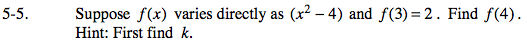### Home > PC > Chapter 5 > Lesson 5.1.1 > Problem5-5

5-5.Write the direct variation equation.

Substitute the given point to find k.

y = k(x2 − 4)

2 = k(32 − 4)
2 = k(5)

$k=\frac{2}{5}$

Write the function with the k value.

Find f(4).

$y=\frac{2}{5}(x^2-4)$

$y=\frac{2}{5}(4^2-4)$

$y=\frac{2}{5}(12)$

$y=\frac{24}{5}$